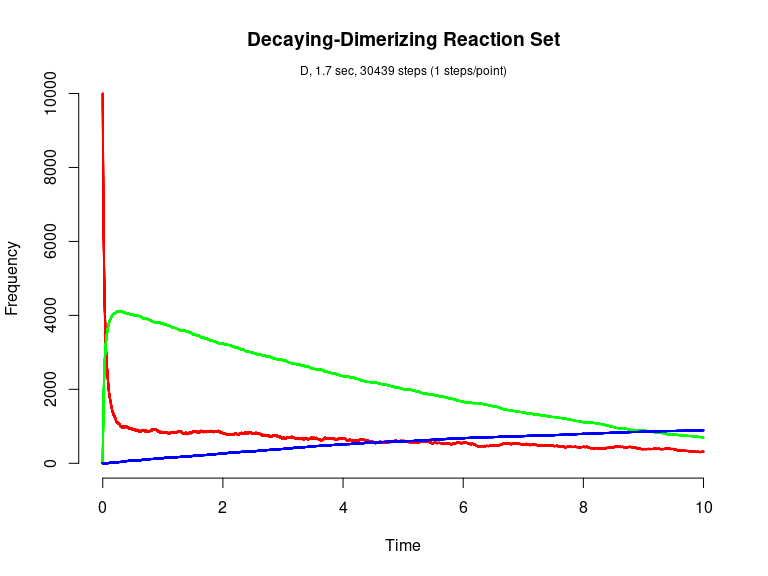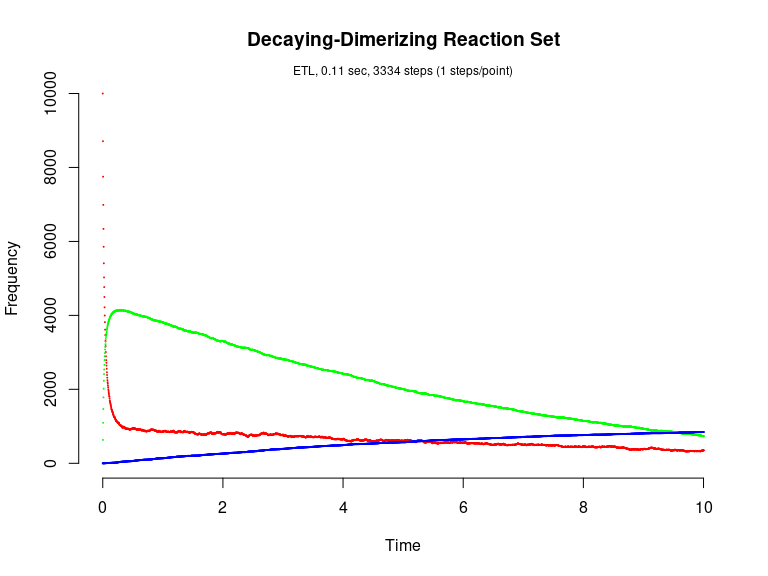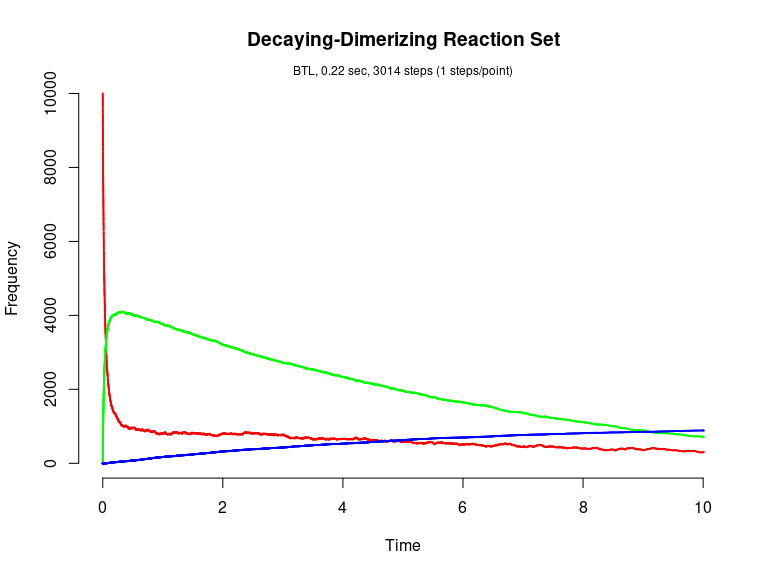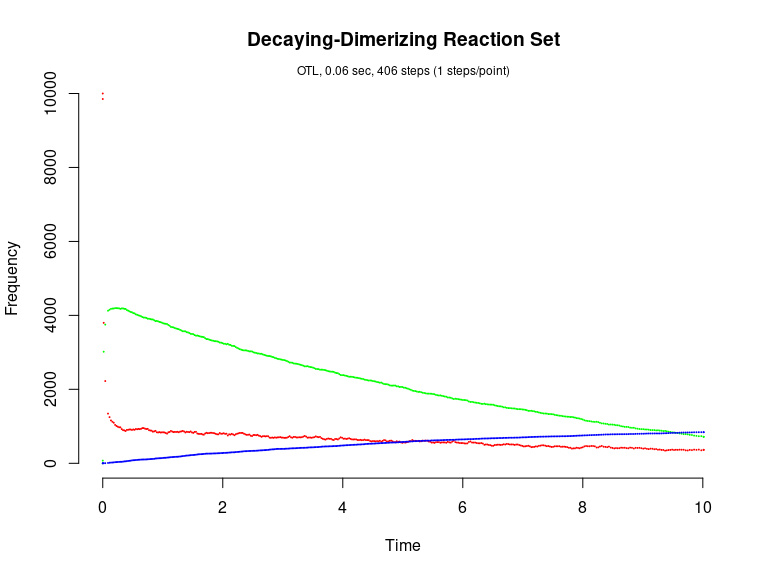# Decaying-Dimerization Reaction Set (Gillespie, 2001)

The Decaying-Dimerization Reaction Set consists of three species and four reaction channels.

      S1 --c1--> 0
S1 + S1 --c2--> S2
S2 --c3--> S1 + S1
S2 --c4--> S3

library(GillespieSSA)

Define parameters

parms <- c(c1=1.0, c2=0.002, c3=0.5, c4=0.04)
tf <- 10                                       # Final time
simName <- "Decaying-Dimerizing Reaction Set"  # Name

Define initial state vector

x0 <- c(s1=10000, s2=0, s3=0)

Define state-change matrix

nu <- matrix(c(-1, -2, +2,  0,
0, +1, -1, -1,
0,  0,  0, +1),
nrow=3,byrow=TRUE)

Define propensity functions

a  <- c("c1*s1", "c2*s1*s1", "c3*s2", "c4*s2")

Run simulations with the Direct method

set.seed(1)
out <- ssa(
x0 = x0,
a = a,
nu = nu,
parms = parms,
tf = tf,
method = ssa.d(),
simName = simName,
verbose = FALSE,
consoleInterval = 1
)
ssa.plot(out, show.title = TRUE, show.legend = FALSE)Run simulations with the Explict tau-leap method

set.seed(1)
out <- ssa(
x0 = x0,
a = a,
nu = nu,
parms = parms,
tf = tf,
method = ssa.etl(tau = 0.003),
simName = simName,
verbose = FALSE,
consoleInterval = 1
)
ssa.plot(out, show.title = TRUE, show.legend = FALSE)Run simulations with the Binomial tau-leap method

set.seed(1)
out <- ssa(
x0 = x0,
a = a,
nu = nu,
parms = parms,
tf = tf,
method = ssa.btl(),
simName = simName,
verbose = FALSE,
consoleInterval = 1
)
ssa.plot(out, show.title = TRUE, show.legend = FALSE)Run simulations with the Optimized tau-leap method

set.seed(1)
out <- ssa(
x0 = x0,
a = a,
nu = nu,
parms = parms,
tf = tf,
method = ssa.otl(),
simName = simName,
verbose = FALSE,
consoleInterval = 1
)
ssa.plot(out, show.title = TRUE, show.legend = FALSE)